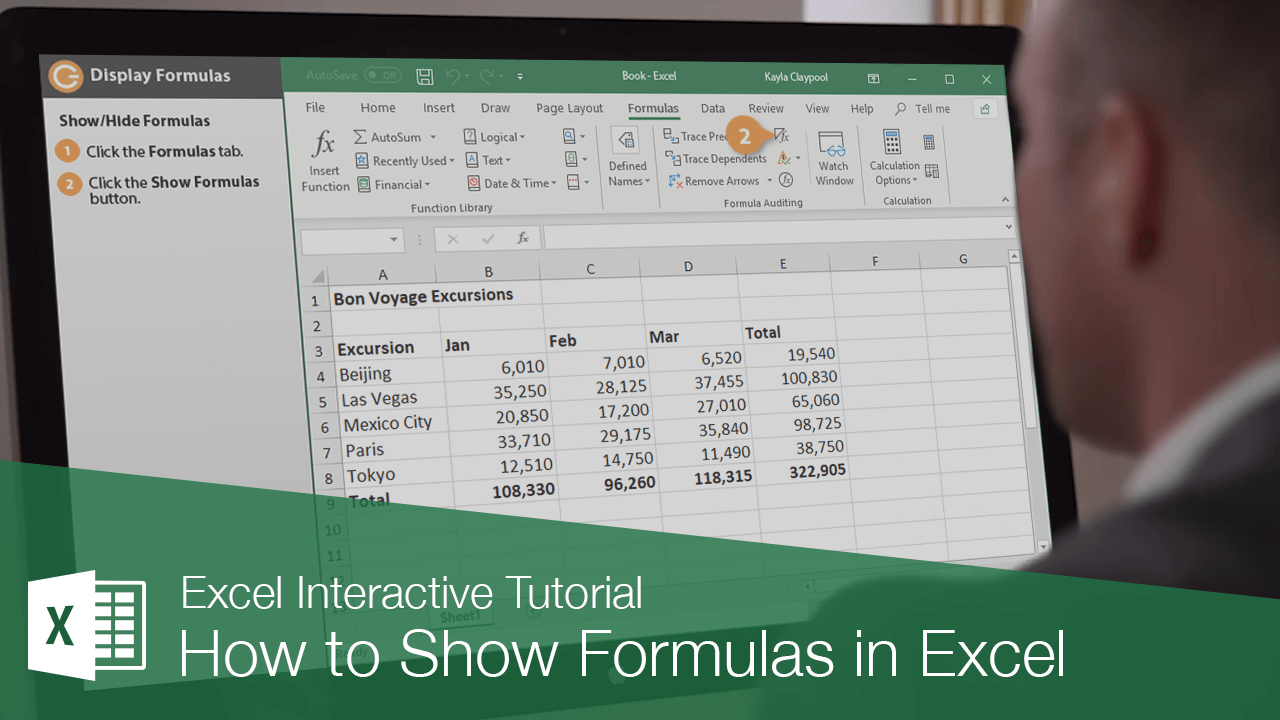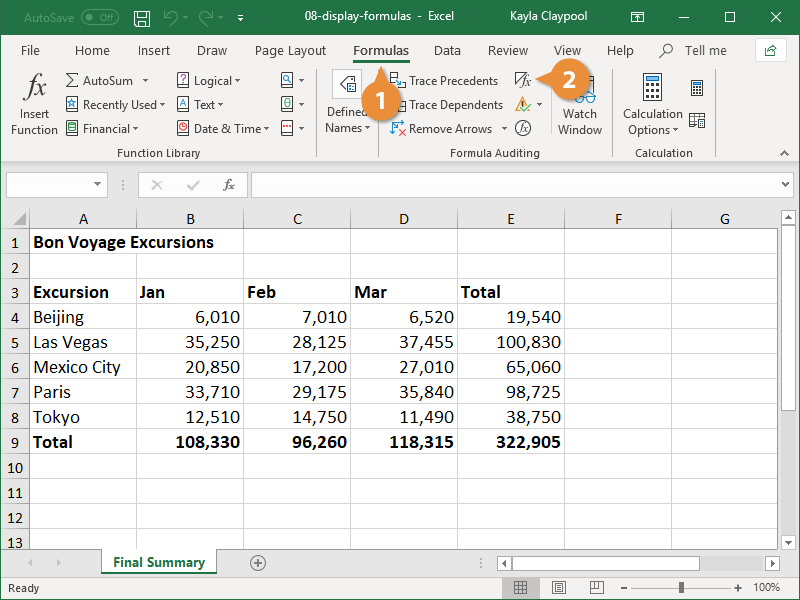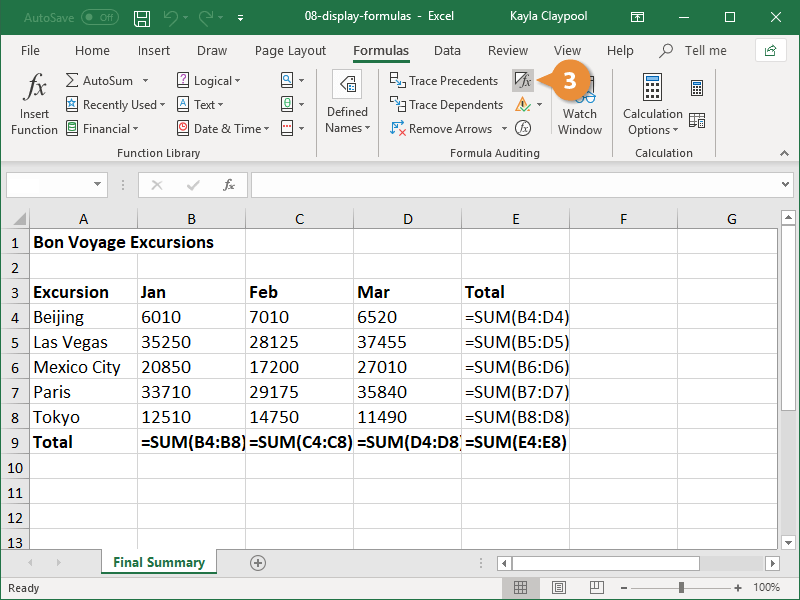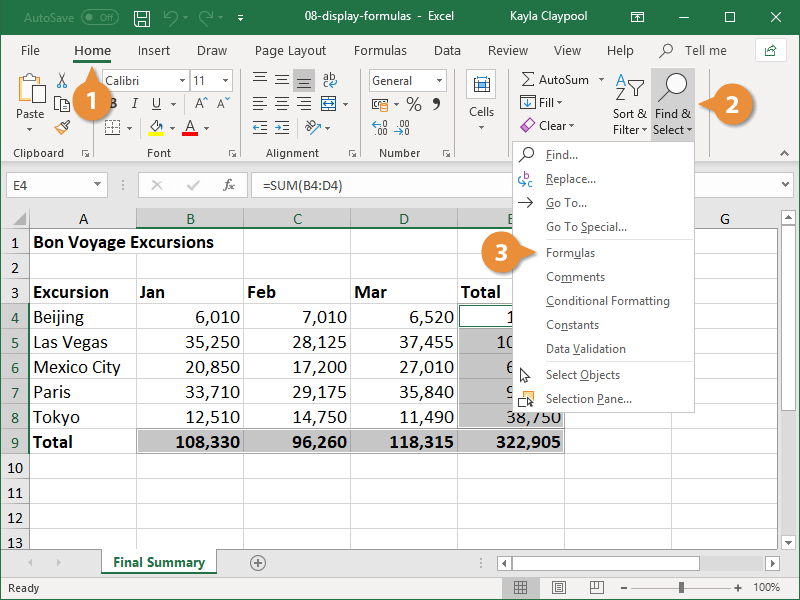How to Show Formulas in Excel | CustomGuide# How to Show Formulas in Excel

How to Display Formulas in ExcelYou can better understand what’s going on in your workbooks by showing and highlighting all the formulas in a sheet.

### Show/Hide Formulas

By default, Excel displays the results of formulas in the worksheet instead of showing the actual formulas. However, you can choose to have Excel display the formulas so you can see how they’re put together.

1. Click the Formulas tab.
2. Click the Show Formulas button.Formulas are displayed in the worksheet and the columns widen to accommodate the formulas, if necessary.

If you display formulas and then select a cell that contains a formula, colored lines appear around cells that are referenced by the formula.

3. Click the Show Formulas button again to hide the formulas.Formulas are no longer displayed and the columns return to their original sizes.

### Highlight Formulas

If you don’t want to see the actual formulas, but want to know which cells contain them, highlight cells with formulas instead.

1. Click the Home tab.
2. Click the Find & Select button.
3. Select Formulas.Any cells that contain a formula are highlighted; however, this doesn’t change the cell formatting. When you click any other cell in the worksheet, the highlighted cells are unselected.